# Texas Go Math Grade 4 Lesson 9.4 Answer Key Estimate Quotients Using Compatible Numbers

efer to our Texas Go Math Grade 4 Answer Key Pdf to score good marks in the exams. Test yourself by practicing the problems from Texas Go Math Grade 4 Lesson 9.4 Answer Key Estimate Quotients Using Compatible Numbers.

## Texas Go Math Grade 4 Lesson 9.4 Answer Key Estimate Quotients Using Compatible Numbers

Essential Question

How can you use compatible numbers to estimate quotients?
we can use the compatible numbers to estimate quotient.
we can choose the number that is close to the dividend and easy to divide by the divisor.

Unlock the Problem

A horse’s heart beats 132 times in 3 minutes. About how many times does it beat in 1 minute?

You can use compatible numbers to estimate quotients.

Compatible numbers are numbers that are easy to compute mentally.

• Will a horse’s heart beat more or fewer than 132 times in 1 minute?
Yes
Explanation:
132 ÷ 3 = 44
44 times in 1 minute
compatible numbers can use compatible numbers to estimate quotients
if compatible number 120
120 ÷ 3 = 40
if compatible number 150
150 ÷ 3 = 50

• What operation will you use to solve the problem?
Division   ‘÷’
Explanation:
Division operation will you use to solve the problem.
The division is a method of distributing a group of things into equal parts.

Example 1

Estimate. 132 ÷ 3
STEP 1 Find a number close to 132 that divides easily by 3. Use basic facts.
12 ÷ 3 is a basic fact. 120 divides easily by 3.
15 ÷ 3 is a basic fact. 150 divides easily by 3.
Think: Choose 120 because it is closer to 132.
120 ÷ 3 = 40

STEP 2 Use place value.
120 = ___________ tens
12 ÷ 3 = ___________
12 tens ÷ 3 = __________ tens
120 ÷ 3 = __________
120 = 12 tens
12 ÷ 3 = 4
12 tens ÷ 3 = 4 tens
120 ÷ 3 = 40

So, a horse’s heart beats about ___________ times a minute
Explanation:
120 = 12 tens
12 ÷ 3 = 4
12 tens ÷ 3 = 4 tens
120 ÷ 3 = 40

Example 2 Use compatible numbers to find two estimates that the quotient is between. 1,382 ÷ 5

STEP 1 Find two numbers close to 1,382 that divide easily by 5.
___________ ÷ 5 is a basic fact.
1,000 divides easily by 5.

___________ ÷ 5 is a basic fact.
1500 divides easily by 5.

1,382 is between ___________ and ___________.
So, 1,382 ÷ 5 is between ___________ and ___________.
10 ÷ 5 is a basic fact.
1,000 divides easily by 5.
15 ÷ 5 is a basic fact.
1500 divides easily by 5.
1,382 is between 1000 and 1500.
So, 1,382 ÷ 5 is between 1000 and 1500.

STEP 2 Divide each number by 5. Use place value. 1,000 ÷ 5
___________ hundreds ÷ 5 = ___________ hundreds, or ___________ 1,500 ÷ 5
___________ hundreds ÷ 5 = ___________ hundreds, or ___________
15 hundreds ÷ 5 = 3 hundreds, or 1,500 ÷ 5
10 hundreds ÷ 5 = 2 hundreds, or 1000 ÷ 5
Explanation:
10 ÷ 5 is a basic fact.
1,000 divides easily by 5.
15 ÷ 5 is a basic fact.
1500 divides easily by 5.
1,382 is between 1000 and 1500.
So, 1,382 ÷ 5 is between 1000 and 1500.

Math Talk

Mathematical Processes
Explain which estimate you think is more reasonable.
Basic fact method of thinking is more reasonable.
To find a value that is close enough to the right answer, usually with some thought or calculation involved is called Estimate.

Share and Show

Estimating Quotients 4th Grade Question 1.
Estimate. 1,718 ÷ 4
__________ is close to 1,718.
__________ is close to 1718.
Choose 1,600 because ___________________

Think: What number close to 1,718 is easy to divide by 4?
What basic fact can you use? __________ ÷ 4
What basic fact can you use? __________ ÷ 4

16 ÷ 4 = ___________
1600 ÷ ___________ = ___________
1,718 ÷ 4 is about ___________
Estimate. 1,718 ÷ 4
1600 is close to 1,718.
1800 is close to 1718.
Choose 1,600 because ___________________
Think: What number close to 1,718 is easy to divide by 4?
What basic fact can you use? 16 ÷ 4
What basic fact can you use? 1600 ÷ 4
16 ÷ 4 = 4
1600 ÷ 4 = 400
1,718 ÷ 4 is about 400
Explanation:
STEP 1 Find a number close to 1,718 that divides easily by 4. Use basic facts.
STEP 2 Divide each number by 4. Use place value. 1,000 ÷ 4

Math Talk

Mathematical Processes
Explain how your estimate change if the problem were 1,918 ÷ 4
Estimate. 1,918 ÷ 4
1,800 is close to 1,918.
2,000 is close to 1,918.
Choose 2,000 because compatible number
Explanation:
STEP 1 Find a number close to 1,918 that divides easily by 4. Use basic facts.
STEP 2 Divide each number by 4. Use place value. 1,000 ÷ 4
Think: What number close to 1,918 is easy to divide by 4?
What basic fact can you use? 20 ÷ 4
What basic fact can you use? 2,000 ÷ 4
20 ÷ 4 = 5
2,000 ÷ 4 = 500
1,918 ÷ 4 is about 500

Use compatible numbers to estimate the quotient.

Question 2.
455 ÷ 9
Estimate. 450 ÷ 9
450 is close to 455.
Choose 450 because the compatible number
Explanation:
STEP 1 Find a number close to 455 that divides easily by 9. Use basic facts.
STEP 2 Divide each number by 9. Use place value. 100÷ 9
Think: What number close to 455 is easy to divide by 9
What basic fact can you use? 45 ÷ 9
What basic fact can you use? 450 ÷ 9
45 ÷ 9 = 5
450 ÷ 9 = 50
455 ÷ 9 is about 50

4th Grade Compatible Numbers Question 3.
1,509 ÷ 3
Explanation:
Estimate. 1,509 ÷ 3
1500 is close to 1,509.
Choose 1500 because the compatible number
Think: What number close to 1500 is easy to divide by 3
What basic fact can you use? 15 ÷ 3
What basic fact can you use? 1500 ÷ 3
15 ÷ 3 = 5
1500 ÷ 3 = 500
1500 ÷ 3 is about 500

Question 4.
176 ÷ 8
Explanation:
Estimate. 176 ÷ 8
160 is close to 176.
Choose 160 because compatible number
Think: What number close to 160 is easy to divide by 8
What basic fact can you use? 16 ÷ 8
What basic fact can you use? 160 ÷ 8
16 ÷ 8 = 2
160 ÷ 8 = 20
176 ÷ 8 is about 20

Question 5.
2,795 ÷ 7
Explanation:
Estimate. 2,795 ÷ 7
2,800 is close to 2,795.
Choose 2800 because compatible number
Think: What number close to 2800 is easy to divide by 7
What basic fact can you use? 28 ÷ 7
What basic fact can you use? 2800 ÷ 7
28 ÷ 7 = 4
2800 ÷ 7 = 400
2,795 ÷ 7 is about 400

Problem Solving

Algebra Estimate to compare. Write <, >, or =.

Question 6.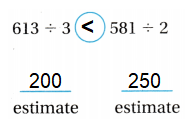Explanation:
Estimate. 613 ÷ 3
200 is close to 204.
Estimate. 581÷ 2
250 is close to 290.
So, 200 is less than 250.

Question 7.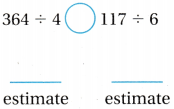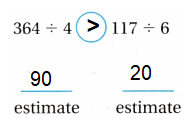Explanation:
Estimate. 364 ÷ 4
90 is close to 91.
Estimate. 117 ÷ 6
20 is close to 21.
So, 90 is greater than 20

Estimating Quotients Grade 4 Question 8.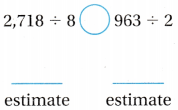Explanation:
Estimate. 2,718 ÷ 8
300 is close to 339.
Estimate. 963 ÷ 2
450 is close to 481.
So, 300 is less than 450

Question 9.
Mr. Morrison put 4,689 miles on his car the first 6 months he owned it. if he drove the same number of miles each month, about how many miles did he drive in one month? Use compatible numbers to find two estimates that the quotient is between.
Explanation:
Estimate. 4,689 ÷ 6
4,800 is close to 4,689.
Choose 4800 because compatible number
Think: What number close to 4800 is easy to divide by 6
What basic fact can you use? 48 ÷ 6
What basic fact can you use? 4,800 ÷ 6
48 ÷ 6 = 8
4800 ÷ 6 = 800
4,689 ÷ 6 is about 800

Question 10.
Multi-Step Leigh wants to buy a bike for $429 and a helmet for$38. If she saves S8 a week, about how many weeks will it take her to save the money to buy the bike and helmet?
$429 +$38 = \$467
Explanation:
Estimate. 467 ÷ 8
480 is close to 467.
Choose 480 because compatible number
Think: What number close to 480 is easy to divide by 8
What basic fact can you use? 48 ÷ 8
What basic fact can you use? 480 ÷ 8
48 ÷ 8 = 6
480 ÷ 8 = 60
480 ÷ 8 is about 60

Problem Solving

Use the table for 11-14.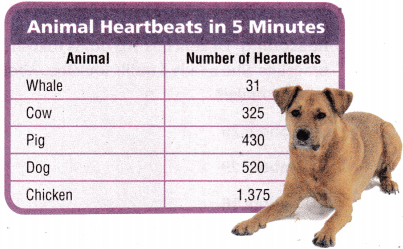Question 11.
About how many times does a chicken’s heart beat in 1 minute?
275 times does a chicken’s heart beat in 1 minute
Explanation:
Number of heart beats of chicken in 5 minutes
1375 ÷ 5 = 275
275 times does a chicken’s heart beat in 1 minute

Question 12.
Multi-Step About how many 6 times does a cow’s heart beat in 2 minutes?
Explanation:
Cow heart beat in 5 minutes
325 ÷ 5
65 times per minute
65 x 2 =130
130 ÷ 6 = 21.6

Question 13.
H.O.T. About how many times faster does a cow’s heart beat than a whale’s?
Cow heart beat is about more than 3 times faster than whale.
Explanation:
Cow heart beat in 5 minutes
325 ÷ 5 = 21.6
whale heart beat in 5 minutes
31 ÷ 5 = 6.2
Cow heart beat is about more then 3 times faster then whale

Question 14.
Write Math What’s the Question? The answer is about 100 beats in 1 minute.
Which animal heart beat is more then 100 times in minute?
Explanation:
Dog heart beat in 5minutes
520 ÷ 5 =104
So, Dog heart beat is 100 in 1 minute.

Estimate Quotients Using Compatible Numbers Lesson 9.4 Answer Key Question 15.
Use Tools Jamie and his two brothers divided a package of 126 toy cars equally. About how many cars did each of them receive?
42 toy cars
Explanation:
Jamie and his two brothers divided a package of 126 toy cars equally.
Number of cars each of them receive
126 ÷ 3 = 42

Question 16.H.O.T. Multi-Step Martha had 154 stamps and her sister had 248 stamps. They combined their collections and put the stamps in an album. If they put 8 stamps on each page, about how many pages would they need?
Explanation:
They combined their collections and put the stamps in an album.
154 + 248 = 402
If they put 8 stamps on each page,
Total pages they need
400 ÷ 8 = 50

Question 17.
Reasoning Dakota has 105 quills that she will use to make bracelets. She needs 4 quills for each. About how many bracelets can she make?
Option (D)
Explanation:
Dakota has 105 quills that she will use to make bracelets.
She needs 4 quills for each.
Number of bracelets she can make
105 ÷ 4

Question 18.
Nick and his three friends divided 252 cards equally. About how many cards did each of them receive?
Option (C)
Explanation:
Nick and his three friends divided 252 cards equally.
Number of cards each of them receive
252 ÷ 4 = 63

Question 19.
Multi-Step A carpenter has 168 doorknobs in his workshop. of those doorknobs, 96 are round and the rest are square. If he wants to place 7 square doorknobs in each bin, about how many bins would he need?
Explanation:
A carpenter has 168 doorknobs in his workshop,
of those doorknobs, 96 are round and the rest are square.
168 – 96 = 72
If he wants to place 7 square doorknobs in each bin,
Total bins he need
72 ÷ 7 = about 10

TEXAS Test Prep

Question 20.
A monkey’s heart beats 1,152 times in 6 minutes. Which is the best estimate of the number of times the monkey’s heart beats in 1 minute?
Option(B)
Explanation:
A monkey’s heart beats 1,152 times in 6 minutes.
The best estimate of the number of times the monkey’s heart beats in 1 minute
1,152 ÷ 6 = 192
Estimate: 200

### Texas Go Math Grade 4 Lesson 9.4 Homework and Practice Answer Key

Use compatible numbers to estimate the quotient.

Question 1.
486 ÷ 6
Explanation:
Compatible numbers are close in value to the actual numbers that make estimating the answer and computing problems easier.
486 ÷ 6
480 ÷ 6 = 80

Question 2.
2,239 ÷ 7
Explanation:
Compatible numbers are close in value to the actual numbers that make estimating the answer and computing problems easier.
2,239÷ 7
2,100 ÷ 7 = 300

Question 3.
2,930 ÷ 4
Explanation:
Compatible numbers are close in value to the actual numbers that make estimating the answer and computing problems easier.
2,940 ÷ 4
2,800 ÷ 4 = 700

Question 4.
5,216 ÷ 8
Explanation:
Compatible numbers are close in value to the actual numbers that make estimating the answer and computing problems easier.
5,216 ÷ 8
5,200 ÷ 8 = 650

Question 5.
396 ÷ 7
Explanation:
Compatible numbers are close in value to the actual numbers that make estimating the answer and computing problems easier.
396 ÷ 7
420 ÷ 7 = 60

Question 6.
1,739 ÷ 9
Explanation:
Compatible numbers are close in value to the actual numbers that make estimating the answer and computing problems easier.
1,739÷ 9
1,800 ÷ 9
1,800 ÷ 9 = 200

Estimate to compare. Write <, >, or =.

Question 7.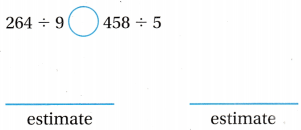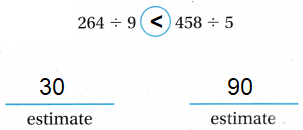Explanation:
Estimate. 264 ÷ 9
30 is close to 29.
Estimate. 458 ÷ 5
90 is close to 91.
So, 30 is less than 90

My Homework Lesson 9.4 Estimate Quotients Answer Key Question 8.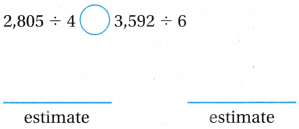Explanation:
Estimate. 2,805 ÷ 4
700 is close to 701.
Estimate. 3,592 ÷ 6
600 is close to 658.
So, 700 is greater than 600

Question 9.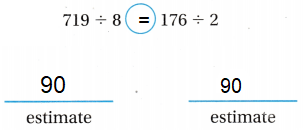Explanation:
Estimate. 719 ÷ 8
90 is close to 89.
Estimate. 176 ÷ 2
90 is close to 88.
So, 90 is equal to 90

Question 10.Explanation:
Estimate. 2,501 ÷ 4
600 is close to 625.
Estimate. 1,486 ÷ 3
500 is close to 495.
So, 600 is greater than 500

Problem Solving

Question 11.
George stacked 126 boxes in a truck in 3 hours. About how many boxes per hour did he stack?
Explanation:
George stacked 126 boxes in a truck in 3 hours.
Number of boxes per hour he stack
basic fact you can use to estimate
126 ÷ 3 = 42
120 ÷ 3 = 40

Question 12.
Casey stacked 441 books equally on 9 shelves. About how many books are on each shelf?
about 50 boos are on each shelf
Explanation:
Casey stacked 441 books equally on 9 shelves.
Number of books on each shelf
basic fact you can use to estimate
441 ÷ 9
450 ÷ 9 = 50

Lesson Check

Question 13.
Marcie vent to the golf course to practice putting. If she made 372 putts in 4 hours, about how many putts did she make each hour?
Option (D)
Explanation:
Marcie made 372 putts in 4 hours,
Number of putts she make each hour
372 ÷ 4
basic fact you can you to estimate
36 ÷ 4 = 9
360 ÷ 4 = 90

Question 14.
‘Wendy and Bother members of her scout troop sold a total of 2,684 boxes of cookies. About how many boxes did each member sell if each scout sold about the same number of boxes?
Option(A)
Explanation:
‘Wendy and Bother members of her scout troop sold a total of 2,684 boxes of cookies.
Total boxes each member sell, if each scout sold about the same number of boxes
basic fact you can use to estimate
25 ÷ 5 = 5
2500 ÷ 5 = 500

Question 15.
Mr. Danielson drove 1,998 miles for his job last week. If he drove about the same number of miles each day, about how many miles did he drive each of the 7 days?
Option(B)
Explanation:
Mr. Danielson drove 1,998 miles for his job last week.
If he drove about the same number of miles each day,
Number of miles he drive each of the 7 days
1998 ÷ 7
basic fact you can use to estimate
21 ÷ 7 = 3
2100 ÷ 7 = 300

Question 16.
Sharon has 572 sheets of construction paper to make autograph books for the business fair. Each book will use 8 sheets of paper. About how many books can Sharon make?
Option(A)
Explanation:
Sharon has 572 sheets of construction paper to make autograph books for the business fair.
Each book will use 8 sheets of paper.
Number of books can Sharon make
572 ÷ 8
basic fact you can use to estimate
56 ÷ 8 = 7
560 ÷ 8 = 70

Question 17.
Multi-Step A total of 8,644 people went to the football game. of those people, 5,100 sat on the home side and the rest sat on the visitor’s side. If they filled 8 equal-sized sections on the visitor’s side, about how many people sat in each of these sections?
option(D)
Explanation:
A total of 8,644 people went to the football game,
of those people, 5,100 sat on the home side and the rest sat on the visitor’s side.
8644 – 5100 = 3544
If they filled 8 equal-sized sections on the visitor’s side,
about how many people sat in each of these sections
3,544 ÷ 8
basic fact to estimate
32 ÷ 8 = 4
320 ÷ 8 = 400

Question 18.
Multi-Step If Cade shoots 275 free throw baskets in 2 hours, about how many can he shoot in 5 hours?